# Linear Differential Equations

Also found in: Dictionary, Medical.
The following article is from The Great Soviet Encyclopedia (1979). It might be outdated or ideologically biased.

## Linear Differential Equations

differential equations of the form

(1) y(n) + p1(x)y(n - 1) + ... + pn(x)y = f(x)

where y = y(x) is an unknown function; y(n), y(n - 1), . . ., y are its derivatives; and p1(x), p2(x), . . ., pn (x) (the coefficients) and/(x) (the free term) are given functions. Equation (1) is said to be linear because the unknown function y and its derivatives enter (1) linearly, that is, the degree of y and its derivatives in (1) is one. If f(x) ≡ 0, then equation (1) is said to be homogeneous; otherwise it is inhomogeneous. The general solution y0 = y0(x) of a homogeneous linear differential equation with continuous coefficients pk(x) can be written as

y0 = C1y1(x) + C2y2(x) + ... + Cnyn(x)

where C1, C2, . . ., Cn are arbitrary constants and y1(x), y2(x), . . ., yn(x) are linearly independent particular solutions. Such n solutions are said to form a fundamental system of solutions. An important result states that n particular solutions are linearly independent if and only if their Wronskian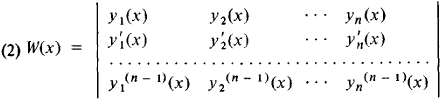is different from zero at at least one point.

The general solution y = y(x) of the inhomogeneous linear differential equation (1) has the form

y = y0 + Y

where y0 = y0(x) is the general solution of the corresponding homogeneous linear differential equation and Y = Y(x) is a particular solution of the given inhomogeneous linear differential equation. The function Y(x) can be found from the formula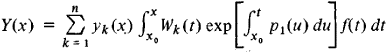where the yk(x) form a fundamental system of solutions of the homogeneous linear differential equation and Wk(x) is the cofactor of the element yk(n - 1)(x) in the Wronskian w(x) given above in (2).

If the coefficients of equation (1) are constant, that is, pk(x) = ak for k = 1, 2, . . ., n, then the general solution of the homogeneous equation is given by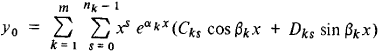Here,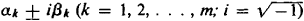) are the roots of the so-called characteristic equation

λn + a1 λn - 1 + ... + an = 0

and the nk are the multiplicities of these roots and Cks and Dks are arbitrary constants.

Example. For the linear differential equation y″′ + y = 0, the characteristic equation has the form λ3 + 1 = 0. Its roots are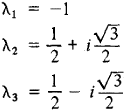Consequently, the general solution of this equation is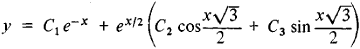Consider a system of n linear differential equations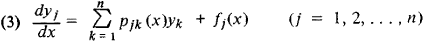The general solution of the homogeneous system of linear differential equations [obtained from (3) by putting all fj(x) ≡ 0] is given by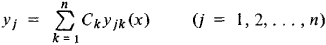where yj1, yj 2, . . ., yjn are linearly independent particular solutions of the homogeneous system (that is, solutions such that the determinant ǀyjk (x)ǀ≠ 0 at at least one point).

In the case of constant coefficients pjk(x) = ajk, particular solutions of the homogeneous system are of the form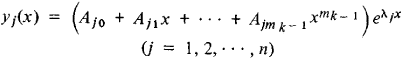where the Ajs are undetermined coefficients and the λk are the roots of the characteristic equation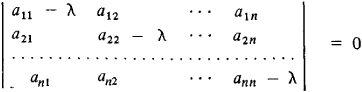with multipliers mk. Here, a complete analysis of all possible cases can be effected by means of the theory of elementary divisors.

The methods of operational calculus are also used to solve linear differential equations and systems of linear differential equations with constant coefficients.

### REFERENCES

Stepanov, V. V. Kurs differentsial’nykh uravnenii, 8th ed. Moscow, 1959.
Smirnov, V. I. Kurs vysshei matematiki. Vol. 2, 20th ed., Moscow, 1967; vol. 3, part 2, 8th ed., Moscow, 1969.
Pontriagin, L. S. Obyknovennye differentsial’nye uravneniia, 3rd ed. Moscow, 1970.
The Great Soviet Encyclopedia, 3rd Edition (1970-1979). © 2010 The Gale Group, Inc. All rights reserved.
References in periodicals archive ?
Chadli, "Solving fuzzy linear differential equations by a new method," Annals of Fuzzy Mathematics and Informatics, vol.
In the value distribution theory of entire functions, Bruck's conjecture  is still an open question, and it has close relation to the following nonhomogeneous complex linear differential equation,
 obtained the Hyers-Ulam stability of a linear differential equation using Laplace transforms.
Massera and J.J.Schaffer: Linear Differential Equations and Function Spaces, Academic Press, New-York, 1966.
Annex: Solving Linear Differential Equations By Elementary Solutions
However, this will require the transition to other (i.e., different from the current ones--see above) algorithms of economic activity, which will be described with linear differential equations.
The Lagrange method for variation of constants applies to linear differential equations of higher order.
Svec, On various properties of the solutions of third- and fourth-order linear differential equations, in Differential Equations and Their Applications, Proceedings of a Conference Held in Prague, September 1962, Academic Press, NewYork, 1963, pp.
Real systems are more complex and need non linear differential equations, generally not easy to solve.
systems of n-th order linear differential equations: (eight hours)

Site: Follow: Share:
Open / Close